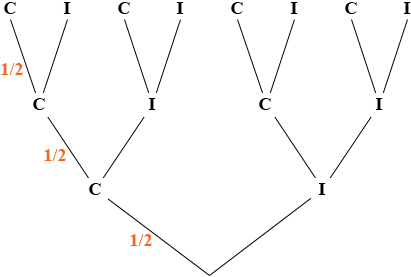A person is given 3 true or false questions. They have no idea of the answers. What is the probability of the person getting them all correct? Could you show me a tree for this. It is a 5th grade question. I think it is 50% but not sure. Thank you for your help. Larry Hi Larry, I think of the tree in three layers. First you answer the first question and get it correct (C) or incorrect (I), next you answer the second question and get it correct (C) or incorrect (I) and finally you answer the third question and get it correct (C) or incorrect (I). Here is my tree.Question one is true or false and the person is guessing, so the probability he guesses the correct answer is 1/2. Question two is true or false and the person is guessing, so the probability he guesses the correct answer is 1/2. Question three is true or false and the person is guessing, so the probability he guesses the correct answer is 1/2. Thus the probability that he gets the first question correct and the second question correct and the third question correct is 1/21/21/2 = 1/8. Penny